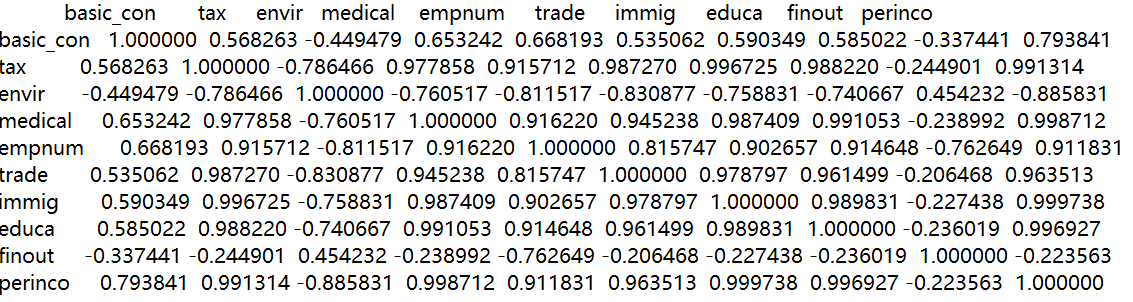•5星
111.52MB qq_28239511 2021-03-17 13:04:53
•5星
503KB weixin_45741872 2021-07-16 11:12:33
•5星
1.41MB weixin_54707168 2021-06-18 16:57:14
•5星
1.34MB dxh_wxx 2021-07-20 09:11:12
•5星
1.2MB u013883025 2021-06-28 16:29:42
•5星
3.3MB weixin_44510615 2021-06-22 10:50:36
•5星
157KB qq_41934573 2021-05-21 23:17:41
•5星
241KB weixin_54707168 2021-06-18 15:22:43
•5星
387KB weixin_44510615 2021-06-23 18:04:15
•5星
294KB weixin_47723732 2021-06-30 18:29:05
• python对excel数据分析常用功能（一文学会如何用Python实现excel基础功能） python excel 数据分析

python对excel数据分析常用功能 （一文学会如何用Python实现excel基础功能） 本文主要应用pandas包完成，先加载pandas包 import pandas as pd 1、excel数据读取 data = pd.read_excel(r"C:\\Users\\Desktop\\test....

python实现excel数据分析常用功能

（一文学会如何用Python实现excel基础功能）

本文主要应用pandas包完成，先加载pandas包

import pandas as pd

1、excel数据读取

路径采用 “\ \” 分隔符可以增加可读性

带有变量的数据载入
day = 6.15
data = pd.read_excel(r"C:\\Users\\Desktop\\" + day + "test.xlsx")
#载入该路径下文件名为：6.15test 的表格

2、数据处理

将导入数据变成数据框，方便调用

data = pd.DataFrame(data)

替换空值

data.fillna(value='none_value', inplace=True)

参数释义：

参数名释义
value=接上想要替换空缺值的内容
inplace=True为在原数据上替换空缺值

去除空值

names = [data['name']_ for data['name']_ in data['name'] if data['name']_ == data['name']_]

去重

data.drop_duplicates(subset='name', keep='first')

参数释义：

参数名释义
subset=接上想要去重的列名
keep=first为保留重复数据的第一个，last为保留重复数据的最后一个，FALSE为把重复数据全部删除

重新排序索引

data.reset_index(drop=True)

去重之后会有一些索引缺失，以上代码可以重新定义索引

3、数据调用

data1 = data['name']  #调用列名为‘name’的列
data2 = data[['name', 'age']]  #调用列名为‘name’和‘age’的列

4、表格合并

merge函数可以进行表格的横向合并（可替代excel中的VLOOKUP函数）

data = pd.merge(left=data1, right=data2, how='left', left_on='name', right_on='name')

参数名释义
left=放在左边的预合并表
right=放在右边的预合并表
how=’left‘即以左边表关键字为准，’right‘以右边为准，’outer‘以关键字并集为准，’inner‘以关键字交集为准
left_on=指定左边表关键字列
right_on=指定右边表关键字列

concat函数可以进行表格的纵向合并

data = pd.concat([data, data2], axis=0)

参数名释义
[ , ]需要进行合并的表格
axis=0表示纵向合并

5、数据计算

两列对应相加减乘除可以直接计算

data3 = data['age']*data['score']

列中每个数同时加减乘除同一常数，需要先进行列表解析，再进行计算

data4 = pd.Series(data['score'])*5.6
#Series函数对列表进行解析

学会以上规则，再善用循环和条件语句就能轻松利用Python实现excel的基本功能~

展开全文weixin_45519258 2021-06-15 15:16:26
• 作为一个学习用Python进行数据分析的新手来说，通过本文来记录分享一些我在用Python中的pandas、numpy来分析Excel表中数据的数据清洗和整理的工作，目的是熟悉numpy以及pandas基础操作，所有操作利用Excel均可以方便...

作为一个学习用Python进行数据分析的新手来说，通过本文来记录分享一些我在用Python中的pandas、numpy来分析Excel表中数据的数据清洗和整理的工作，目的是熟悉numpy以及pandas基础操作，所有操作利用Excel均可以方便实现。备注：本文中使用的是ipython完成的编辑

数据来源及结构

本文所分析的数据是通过爬虫抓取的微博数据。选取新浪微博为数据平台，选取我国34个省的旅游政务官方微博为研究对象，利用爬虫软件Gooseeker爬取微博信息，包括用户名、粉丝数、开博日期、当月原创微博总数No、当月总微博数N、单条博文的转发数、单条博文的评论数、条博文的点赞数。

爬取的数据表格test.xlsx包括以下几个sheet中，基本表结构见下1、All(所有博文)：所有字段见下：其中有用字段是‘用户名、微博内容、时间、转发数、评论数、点赞数’，共有6585条数据(备注：转发数中的缺失值为“转发”，评论数中的缺失值为“评论”，点赞数中的缺失值为“赞”)：2、sf(各省信息表，此表中：省份名完整)3、sfwibo (此表记录的是各个微博账号对应的省份名，省份名不完整，已知各个省份名只有前两个字完整)4、base_info2(爬取的微博账户的相关信息)(备注：此处的“昵称”与sfweibo中的“微博用户名”等同)本文思路：

Step1：对于All表：1)取出有用字段。2)处理缺失值。3)数据透视

Step2：对于sf 和sfweibo 表：1)以省份名做数据连接成sf_sfweibo。2)并与All表做数据连接sf_sfweibo_All。

Step3：对于base_info表：1)与sf_sfweibo_All做数据连接 2)计算h值 3)处理数据4)计算相关性

Step4：导出最后结果到一个Excel文件中，完成数据处理。、

数据处理过程：

step1 ：

# -*- coding=utf-8 -*-

#导入需要的包

from__future__import division

import numpy as np

import pandas as pd

import matplotlib.pyplot as plt

from numpy.randomimport randn

from pandasimport Series, DataFrame

from datetimeimport datetime

import xlrd, openpyxl

xlsx_file = pd.ExcelFile('test1.xlsx')

All = xlsx_file.parse('All')

1)取出有用字段

#删掉表格中的无用列，保留有用列

d1 = All.drop(All.columns[:11], axis=1, inplace = False)

All = d1.drop(d1.columns[-1], axis=1, inplace = False)

#显示表格前五行#查看去重未处理前表中记录长度

len(All)#获取到重复的行的行号的前20个

All[All.duplicated()==True].index[:20]#删除掉重复的行，在原值上直接修改

All.drop_duplicates(inplace=True)

len(All)#通过运行结果，可以发现确实删掉了，当前记录条数为6159条2)处理缺失值

#处理缺失值，先获取该列，将列中的"转发”、"评论"、"赞"替换掉

#为什么不在读取表格的时候直接设置呢？因为如果一开始就设置替换，会导致整张表中的"转发”、"评论"、"赞"均会被替换掉，造成信息损失

All[u'转发数'][All[u'转发数']==u'转发'] = '0'

All[u'评论数'][All[u'评论数']==u'评论'] = '0'

All[u'点赞数'][All[u'点赞数']==u'赞'] = '0' #等价于# All[u'点赞数'].replace(u'赞','0')3)数据透视

All.describe()备注：出现这个结果说明了数据类型不是数值型

#查看表中各个列的数据类型

All.dtypes#为了能进行数据透视，需要将对应列的数据类型转换成数值型

#将DataFrame表中的某列数据进行转换类型

All[u'转发数']=All[u'转发数'].astype('int64')

All[u'评论数'] = All[u'评论数'].astype('int64')

All[u'点赞数'] = All[u'点赞数'].astype('int64')

All.describe()#查看表中各个列的数据类型

All.dtypes#将预处理过的表保存到All.xlsx中

All.to_excel('All.xlsx',index=False)

#数据透视表

All_pivot= All.pivot_table(values=[u'转发数',u'评论数',u'点赞数',u'微博内容'],index=[u'用户名'],\

aggfunc={u'转发数':np.sum,u'评论数':np.sum,u'点赞数':np.sum,u'微博内容':np.size})

#给该列换名称

All_pivot.rename(columns={u'微博内容':u'当月总微博数'},inplace=True)

All_pivot#将完成的透视表保存

All_pivot.to_excel('All_pivot.xlsx')

step2

1)以省份名做数据连接成sf_sfweibo

#读取test1.xlsx中的sf表

sf = xlsx_file.parse('sf')#读取test1.xlsx中的sfweibo表

sfweibo = xlsx_file.parse('sfweibo')#通过上面的表格输出可以看出，要想将sf和sfweibo进行连接，需要对sf以及sf微博中的“省份名”

#但是，由于sfweibo中的省份名是不完整的，已知名称中肯定包含省份中的前两个字，为此，需要对两个表格切割后，进行连接

sf[u'省份前两字'] = np.nan

for iin range(len(sf[u'省份名'])):

sf[u'省份前两字'][i] = sf[u'省份名'][i][:2]

sfweibo[u'省份前两字'] = np.nan

for iin range(len(sfweibo[u'省份名'])):

sfweibo[u'省份前两字'][i] = sfweibo[u'省份名'][i][:2]

#显示表格的前五行#显示表格的前五行#保存数据

sf.to_excel('sf.xlsx',index=False)

sfweibo.to_excel('sfweibo.xlsx',index=False)

#连接两表

sf_sfweibo = sf.merge(sfweibo,on=u'省份前两字')#获取连接后表格中需要的字段名，并重新排列

sf_sfweibo1 = sf_sfweibo.iloc[:,[4,1,2]]#存储连接后的表

sf_sfweibo1.to_excel('sf_sfweibo.xlsx',index=False)

2)并与All表做数据连接sf_sfweibo_All

#连接sf_sfweibo和All_pivot两表

sf_sfweibo = sf_sfweibo1

sf_sfweibo_All_pivot =pd.merge(sf_sfweibo,All_pivot,left_on=u'微博用户名',right_on=u'用户名',right_index=True)

#显示连接后的表格的前五行#将连接后的表进行存储

sf_sfweibo_All_pivot.to_excel('sf_sfweibo_All_pivot.xlsx',index=False)

step3:

1)与sf_sfweibo_All做数据连接

#处理爬取的用户的基本信息表base_info

base = xlsx_file.parse('base_info')#将base表与sf_sfweibo_All_pivot进行连接

sf_sfweibo_All_pivot_base = base.merge(sf_sfweibo_All_pivot,left_on=u'昵称',right_on=u'微博用户名')

ssapb = sf_sfweibo_All_pivot_base #名称太长，换个名称#替换某列的名字

ssapb.rename(columns={u'当月总微博数_x':u'当月总微博数'},inplace=True)

#删除其中的多余列

ssapb = ssapb.drop([u'昵称',u'当月总微博数_y'],axis=1)

#读取第一行的数

ssapb.iloc#添加一列(当月原创数=当月总微博数-当月转发数)

ssapb[u'当月原创数'] = ssapb[u'当月总微博数']-ssapb[u'当月转发数']

#将某列同时与某段字符串连接，通过观察网页可以发现这是网址的特点

allfix = "?profile_ftype=1&is_all=1#_0"

ssapb[u'全部博文网址'] = ssapb[u'主页链接']+allfix

#计算出篇均转发/点赞/评论，并添加列

ssapb[u'篇均点赞'] = ssapb[u'点赞数']/ssapb[u'当月总微博数']

ssapb[u'篇均转发'] = ssapb[u'转发数']/ssapb[u'当月总微博数']

ssapb[u'篇均评论'] = ssapb[u'评论数']/ssapb[u'当月总微博数']

#读取表中的第一行数据

ssapb.iloc#存储表格

ssapb.to_excel('ssapb.xlsx',index=False)

2)计算h值

#将All表分组，获取表格的index值

gb = All.groupby(u'用户名')

gb1 = gb.size()

gbindex = gb1.index

print gbindex,gb1#根据h指数的定义，分别计算转发/评论/点赞h指数

#再记录下每个“用户名的最大互动度max(转发+评论+点赞)”

sortAllf = All.sort_values(by=[u'用户名',u'转发数'],ascending=[True,False])

sortAllc = All.sort_values(by=[u'用户名',u'评论数'],ascending=[True,False])

sortAlll = All.sort_values(by=[u'用户名',u'点赞数'],ascending=[True,False])

mm = (sortAllf,sortAllc,sortAlll)

#将计算得到的结果重新存储到一个新的DataFrame中

All_h =pd.DataFrame(np.arange(136).reshape(34,4),columns=['fh','ch','lh','max_hdd'],index=gbindex)

fh=[]

ch=[]

lh=[]

max_hdd = []

for jin range(len(mm)):

for iin gbindex:

tempdf =mm[j][mm[j][u'用户名']==i]

tempdf['hdd'] = tempdf[u'转发数']+tempdf[u'评论数']+tempdf[u'点赞数']

max_hdd.append(tempdf['hdd'].max())

tempdf['numf'] = range(len(tempdf))

if  j==0:

a =len(tempdf[tempdf[u'转发数']>=tempdf['numf']+1])

fh.append(a)

elif  j==1:

b =len(tempdf[tempdf[u'评论数']>=tempdf['numf']+1])

ch.append(b)

else:

c = len(tempdf[tempdf[u'点赞数']>=tempdf['numf']+1])

lh.append(c)

All_h['fh']=fh

All_h['ch']=ch

All_h['lh']=lh

#因为，前面的循环一共循环了三遍，使得All_h重复了3遍，因此只要获取前34位即可

All_h['max_hdd']=max_hdd[:34]

#插入一个综合h指数，该指数是转发/评论/点赞h指数三个的均值

All_h.insert(3,'HS',All_h.iloc[:,:3].mean(1))

#更改列名称

All_h.rename(columns={'fh':u'转发h指数','ch':u'评论h指数',\

'lh':u'点赞h指数','HS':u'综合h指数','max_hdd':u'单篇最大互动度'},inplace=True)#连接ssapb和All_h表格

ssapb_All_h= pd.merge(ssapb, All_h, left_on=u'微博用户名',right_on=u'用户名',right_index=True)

#加一列原创率

ssapb_All_h[u'原创率'] = ssapb_All_h[u'当月原创数']/ssapb_All_h[u'当月总微博数']

ssapb_All_h.iloc#存档

ssapb_All_h.to_excel('ssapb_All_h.xlsx',index=False)

3)计算相关性

#获取原DataFrame中的几列存储到新的DataFrame中，计算综合h指数与其他分指数之间的相关性

f1 = ssapb_All_h.loc[:,[u'综合h指数',u'转发h指数',u'评论h指数',u'点赞h指数']]

#计算f1中各列数据的相关性

corr1 = f1.corr()

#将该相关性结果存档

corr1.to_excel('corr1.xlsx')

corr1#获取原DataFrame中的几列存储到新的DataFrame中，计算综合h指数与其他微博信息之间的相关性

f2 = ssapb_All_h.loc[:,[u'综合h指数',u'转发数',u'评论数',u'点赞数',u'篇均转发',u'篇均评论',u'篇均点赞']]

corr2 = f2.corr()

corr2.to_excel('corr2.xlsx')

corr2#获取原DataFrame中的几列存储到新的DataFrame中，计算综合h指数与其他信息之间的相关性

f3 = ssapb_All_h.loc[:,[u'综合h指数',u'原创率',u'粉丝数',u'微博总数',u'单篇最大互动度']]

corr3 = f3.corr()

corr3.to_excel('corr3.xlsx')

corr3#重新排序列

aa =ssapb_All_h.iloc[:,[8,9,10,5,15,16,0,1,2,3,4,6,14,7,11,12,13,24,20,21,22,23,17,18,19,25]]

aa.to_excel('finally.xlsx')

aa.iloc4)处理数据，处理浮点位数/转成百分位数

#将表中的浮点类型保留至小数点后四为

f = lambda x:'%.4f' % x

aa.ix[:,21:] = aa.ix[:,21:].applymap(f)

aa.ix[:,21:] = aa.ix[:,21:].astype('float64')

aa.iloc#将原创率转成百分比形式

f1 = lambda x :'%.2f%%' %  (x*100)

aa[[u'原创率']]= aa[[u'原创率']].applymap(f1)

aa.to_excel('finally1.xlsx',index=False)

aa.ilocaa.sort_values(by=u'综合h指数', ascending=False, inplace=True)

#按照综合h指数降序排序，添加一个排序位数

aa['rank'] =np.arange(34)+1

#要想得到“综合h指数/排名"的列，需要将aa['rank']和aa[u'综合h指数']进行合并成一列，这就要求必须连接字符串类型

aa['rank'] = aa['rank'].astype('string_')

aa[u'综合h指数'] = aa[u'综合h指数'].astype('string_')

#连接成一列

aa[u'综合h指数/排名'] = aa[u'综合h指数']+'/'+ aa['rank']

aa.ilocstep4

1)存储最终数据

#删除掉一列rank

del aa['rank']

#将该数据类型换回来，换成浮点型

aa[u'综合h指数'] = aa[u'综合h指数'].astype('float64')

aa.to_excel('finally2.xlsx',index=False)

结语：

至此，本次分析操作过程已全部结束，希望这些操作可以新手有一定的参考帮助

展开全文weixin_26854829 2021-02-10 03:35:33

此脚本使用openpyxl库对excel进行操作。

主要功能

此库主要的功能有四个：

1. 读取表：先使用sheetnames字段值取得所有sheet名，然后根据sheet名取得该sheet。然后可以通过cell函数指定row与column取得值（value），如：
sheetnames = workxls.sheetnames
for sheetname in sheetnames:
sheet = workxls.get_sheet_by_name(sheetname)
for i in range(1,workxls.max_row+1):
for j in range(1,workxls.max_column+1):
print(sheet.cell(row=i, column=j).value)

1. 写入表：同样使用的是cell函数，通过设定value值即可更改表中的值，如：
sheet.cell(row=6, column=6, value=6)

1. 保存更改：使用save函数，指定文件名即可保存，如：
workxls.save('result.xlsx')

注意事项：

1. max_row与max_column是取得sheet中数据的最大行与最大列数，但是有时是不准的，因为有的是空行/列，这时，还是手动输入的好。
2. 读取合并格时将最左上的格读成该合并格的值，其他为None。

数据分析

读取excel表中的数据是要进行分析的，这时候首先应该做的是如何保存分析数据，以谁作为键，以谁作为值，是以sheet名分多个部分存储还是合在一个里面。不仅如此，使用字典还是列表等存储方式也会对数据的分析造成影响。

这里我使用字典进行存储，因为主要任务是对数据指标进行排序，然后输出对应排序结果的城市名称，所以我以数据指标作为“大”键，创建字典，然后以“城市:指标值”进行存储。

字典的排序

这个部分花了我很长时间，先说说排序正确格式：

dict={'广州': 0.63385324750839, '深圳': 1, '佛山': 0.4214618485089, '东莞': 0.121729284191413, '北京': 0.472064389109345, '上海': 0.435534382682676, '杭州': 0.497954686878373, '苏州': 0.693492027869304, '南京': 0.543267808505646, '成都': 0}
# 首先要将字典用items函数做成元组，然后用Key=？指定排序的数据，使用reverse决定升序降序
print(sorted(dict.items(),key=lambda dict:dict，reverse=True))

再说说我的历程：
首先我是使用最简单的排序：

sorted(dict，reverse=True)

这个代码在这种情况下输出结果正确：

dict={"3":3,"1":1,"2":2,"4":4}但是在这种情况下结果错误：奇了怪，然后查了很久才查到正确的代码。

示例代码：

sheetnames = workxls.sheetnames
xlsval = dict()

for sheetname in sheetnames:
xlsval[sheetname]=dict()
sheet = workxls.get_sheet_by_name(sheetname)
mrow=17		# 手动输入比使用max_row值更好，因为，鬼知道那个xls的行列到底是多少行，有的没有值也算，离谱
mcol=11

startwr = 19
sheet.cell(row=startwr, column=1,value="指标")
citydict=dict()
for i in range(2, mcol+1):
sheet.cell(row=startwr, column=i, value=sheet.cell(row=2,column=i).value)
citydict[sheet.cell(row=2,column=i).value]=0
startwr+=1

for i in range(3,mrow+1):
xlsval[sheetname][sheet.cell(row=i,column=1).value]=dict()
for j in range(2, mcol+1):
xlsval[sheetname][sheet.cell(row=i, column=1).value][sheet.cell(row=2,column=j).value]=sheet.cell(row=i,column=j).value

sortdict=xlsval[sheetname][sheet.cell(row=i, column=1).value]
# 首先要将字典用items函数，然后用Key=？指定排序的数据，使用reverse决定升序降序。
sortlist = sorted(sortdict.items(),key=lambda sortdict:sortdict,reverse=True)

sheet.cell(row=startwr, column=1, value=sheet.cell(row=i,column=1).value)
for i in range(0,len(sortlist)):
citydict[sortlist[i]]=i+1
colindex=2
for key in citydict.keys():
sheet.cell(row=startwr, column=colindex, value=citydict[key])
colindex+=1
startwr+=1

workxls.save('result.xlsx')

展开全文qq_41392015 2021-06-09 21:38:49
• python从excel中读取数据并进行相关度分析 python

pythonexcel中读取数据并进行相关度分析 # -*- coding: utf-8 -*- """ Created on Fri Nov 27 19:41:32 2020 @author: asus """ import numpy as np import pandas as pd from pandas import read_excel from ...

python从excel中读取数据并进行相关度分析

# -*- coding: utf-8 -*-
"""
Created on Fri Nov 27 19:41:32 2020

@author: asus
"""
import numpy as np
import pandas as pd
from sklearn import preprocessing

pd.set_option('display.max_columns',1000)
pd.set_option('display.width', 1000)
pd.set_option('display.max_colwidth',1000)
data = open('out1.txt','w+')
print(df.corr(),file=data)
data.close()

这里设置阈值1000含义为spyder中结果可能会展示为省略号，设置后会将结果直接输出到txt中
展示如下展开全文weixin_43539576 2020-12-20 12:20:08
• 使用python对excel表格商业数据可视化分析 python 开发语言 后端

visual_eagle 2021-12-14 12:56:21
• python处理excel数据 python

Fengziwen1 2021-07-10 11:08:54
• weixin_31993501 2021-01-28 23:52:56
• 大话 Python：python 操作 excel 系列 -- 数据的提取与筛选 python 数据分析 excel

chengxuyuan_110 2021-03-01 17:16:33
• （Python 篇3）办公报表实用，通过Python读取Excel数据并打印 python excel 数据分析

qq_41973208 2021-03-05 12:36:58
• weixin_35661850 2021-03-17 21:57:39
• weixin_39857174 2021-02-01 03:17:56
• chinacqzgp 2021-02-10 17:07:33
• weixin_34688784 2021-01-13 13:24:00
• 利用Python对Excel数据进行处理 python 数据分析 excel

weixin_54500974 2021-07-07 21:29:58
• weixin_34142735 2021-01-14 14:05:15
• 利用python对EXCEL的提取处理 python excel

weixin_46200756 2021-03-09 08:17:07
• qq_45261963 2021-06-10 17:39:59
• Python简单爬虫+excel数据分析（绘制数据图、排序、查找指定数据） python

qq_3148675740 2021-12-05 16:57:21
• python对Excel数据运用的几种库 python unittest

m0_51709670 2021-08-18 14:36:35
• Python+Excel+SQL，完美解决数据分析问题 人工智能 大数据 数据分析 机器学习 编程语言

weixin_38753213 2021-03-20 00:26:18
• 用Python自动生成Excel数据报表！ python 数据分析 数据可视化 docker 可视化

lemonbit 2021-04-15 00:17:10
• weixin_45914452 2021-02-19 22:39:15
• 大话 Python：python 操作 excel 系列 -- excel 数据分析 python excel 数据分析

chengxuyuan_110 2021-02-26 11:05:31
• weixin_35959694 2021-03-06 06:40:45
• weixin_35682426 2021-02-03 03:30:43
• 用 Python 让 Excel 快速按条件筛选数据 python数据分析

weixin_44026026 2021-01-21 01:29:48
• Echarts基础：用python读取excel数据的网页可视化 echarts python 前端 javascript html5

weixin_43348955 2021-10-22 14:34:51
• weixin_34969621 2021-02-02 23:51:33
• weixin_42123296 2021-03-18 09:52:48...

python对excel数据分析python 订阅